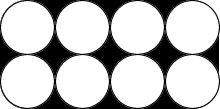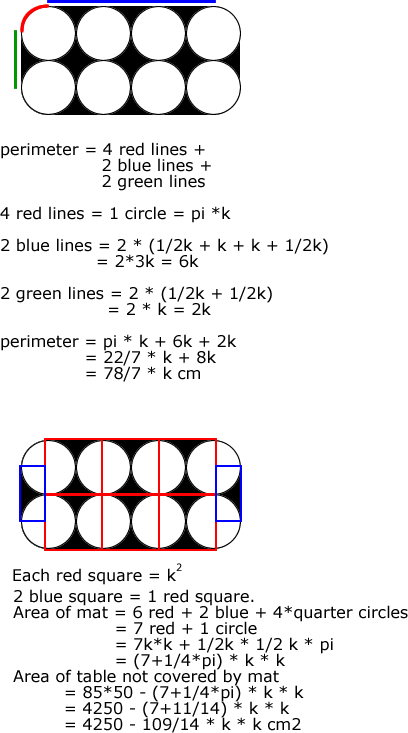# Maths question: Rounded rectangle

The figure below shows a black and white table mat that Mrs Lee bought. The diameter of each circle is k cm. (take pi = 22/7)a) find the perimeter of the table mat in terms of k.

b) Mrs Lee placed the mat on a table which is 0.85m long and 0.5m wide. What is the area of the table that is not covered by the mat in terms of k?

Solution